## Subspace

Let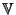be a Real Vector Space (e.g., the real continuous functions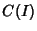on a Closed Interval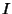, 2-D Euclidean Space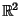, the twice differentiable real functions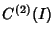on, etc.). Then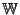is a real Subspace ofifis a Subset ofand, for every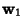,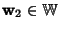and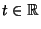(the Reals),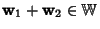and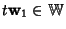. Let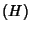be a homogeneous system of linear equations in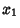, ...,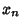. Then the Subset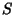of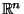which consists of all solutions of the systemis a subspace of.

More generally, let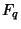be a Field with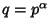, where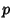is Prime, and let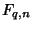denote the-D Vector Space over. The number of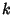-D linear subspaces ofis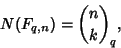where this is the q-Binomial Coefficient (Aigner 1979, Exton 1983). The asymptotic limit is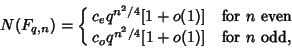where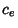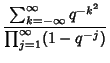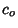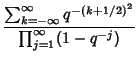(Finch). The casegives the q-Analog of the Wallis Formula.

References

Aigner, M. Combinatorial Theory. New York: Springer-Verlag, 1979.

Exton, H.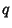-Hypergeometric Functions and Applications. New York: Halstead Press, 1983.

Finch, S. Favorite Mathematical Constants.'' http://www.mathsoft.com/asolve/constant/dig/dig.html